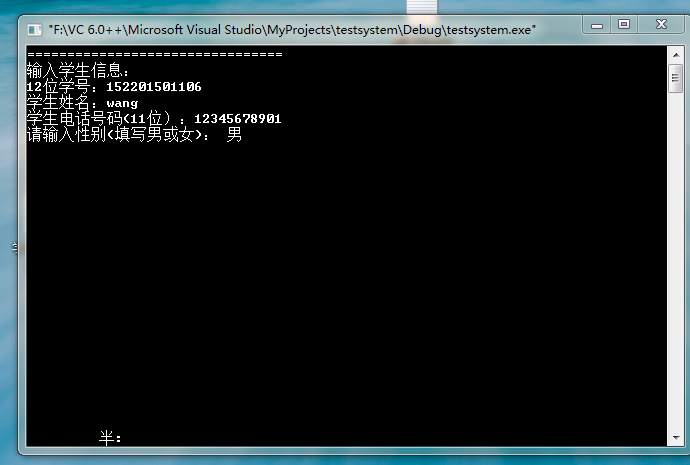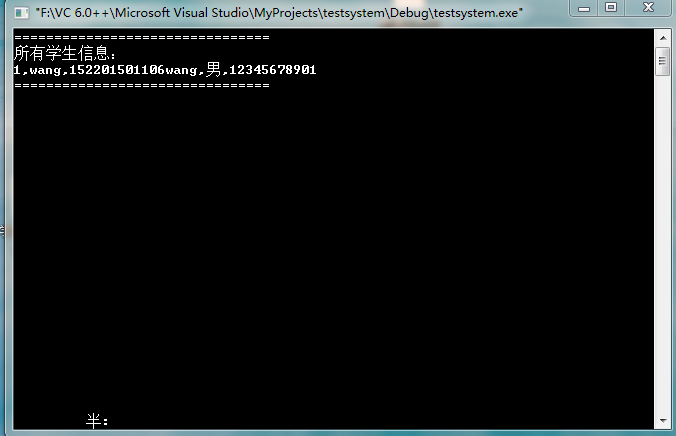C语言 输出时多显示了一个数据#include
#include
#include
#include

int bExit = 0;
int total = 0;
#define Create(the_one) the_one=(Stu *)malloc(sizeof(Stu))
typedef
struct Student
{

``````char num;      // 数字学号
char name; // 姓名
char sex;     // 性别
char telnum;    // 电话号码
struct Student *next;
``````

}Stu;
Stu *the_one, *h, *pr,*last, *end;

//1.添加学生函数
{
int Four = 0;
printf("================================\n");

``````Create(the_one);
the_one->next = NULL;
end = last;          //end指向倒数第二个结点

last->next = the_one;//将the_one放在链表的 最后
last = the_one;      //last指向倒数第一个结点

//输入学号
printf("输入学生信息：\n12位学号：");

while (!Four)
{
gets(the_one->num);
if (strlen(the_one->num) != 12)
printf("输入非法：学号必须是12位，请重新输入!\n学号：");
else
Four = 1;
}

//输入姓名
printf("学生姓名：");
gets(the_one->name);
//输入电话号码
printf("学生电话号码(11位）：");
gets(the_one->telnum);
//请输入性别
printf("请输入性别(填写男或女)： ");
gets(the_one->sex);

//总数+1
total++;
printf("================================\n");
``````

}

//2.查询学生函数
void CHECK()
{
Stu *target, *p;
int fond = 0;
printf("================================\n");
printf("输入要查找学生的学号：\n学号：");
Create(target);
gets(target->num);
for (p = h->next; p; p = p->next)
{
if (!strcmp(p->num, target->num))
{
printf("学号为%s的学生信息：姓名（%s）,性别（%s）,电话号码（%s）\n", p->num, p->name,p->sex, p->telnum);
free(target);
fond = 1;
}
}
if (!fond)
printf("Wrong Student NO.!\n");
printf("================================\n");
}
//3.编辑学生
void EDIT()
{
Stu *target, *p;
int fond = 0;
printf("================================\n");
printf("输入要编辑学生的学号：\n学号：");
Create(target);
gets(target->num);
for (p = h->next; p; p = p->next)
{
if (!strcmp(p->num, target->num))
{
printf("学号为%s的学生信息：姓名（%s）,性别（%s）,电话号码（%s）\n", p->num, p->name,p->sex, p->telnum);
printf("请选择修改项目：\n1：姓名\n2：电话号码\n");
char c;
c = getch();
switch (c)
{
case '1':
printf("输入正确的姓名：");
gets(p->name);
break;
case '2':
printf("输入最新电话号码：");
gets(p->telnum);
break;
}
printf("学号为%s的学生最新信息：姓名（%s）,性别（%s）,电话号码（%s）\n", p->num, p->name,p->sex, p->telnum);

``````        free(target);               // ？？
fond = 1;
}
}
if (!fond)
printf("Not Exit This NO.!\n");
printf("================================\n");
``````

}
//4.删除学生
void DELETE1()
{
void PRINALL();
PRINALL();
Stu *target, *p, *pr;
int fond = 0;
printf("================================\n");
printf("输入要删除学生的学号：（如果删除所有学生信息，请输入0000）\n学号：");
Create(target);
gets(target->num);

``````//  char *zero=&"0000";

if (!strcmp(target->num, "0000"))
{
h->next = NULL;
total = 0;
}
else
{

for (pr = h, p = h->next; p; pr = pr->next, p = p->next)
{
if (!strcmp(p->num, target->num))
{
printf("学号为%s的学生信息已删除\n", p->num);
free(target);
pr->next = p->next;
p->next = NULL;
//               free(p);此句报错
fond = 1;
total--;
}
}
if (!fond)
printf("没有找到学号为%s的学生，请确认学号输入是否正确\n");
}
printf("================================\n");
``````

}
//5.打印全部
void PRINALL()
{
int amounts = 1;
Stu *ap;
printf("================================\n");
printf("所有学生信息：\n");
for (ap = h->next; ap; ap = ap->next)

printf("%d,%s,%s,%s,%s\n",amounts++,ap->name,ap->num,ap->sex,ap->telnum);
printf("================================\n");
}
//6.总人数
void TOTAL()
{
printf("================================\n");
printf("学生总人数：%d\n", total);
printf("================================\n");
}

//菜单函数
{
char order;
printf("================================\n");
printf("| 1.添加学生. |\n");
printf("| 2.查询学生. |\n");
printf("| 3.编辑学生. |\n");
printf("| 4.删除学生. |\n");
printf("| 5.打印全部. |\n");
printf("| 6.总人数. |\n");
printf("| 7.退出. |\n");
printf("================================\n");

``````order = getch();
system("cls");

switch (order)
{
case '1':
break;
case '2':
CHECK();
break;
case '3':
EDIT();
break;
case '4':
DELETE1();
break;
case '5':
PRINALL();
break;
case '6':
TOTAL();
break;
case '7':
bExit = 1;
break;
}
while (!bExit)
{
getch();
system("cls");
}
``````

}
void main()
{
//创建链表头
Create(h);
pr = last = end = the_one = h;
the_one->next = NULL;

``````Menu();
``````

}

1个回答C语言：单链表输出显示问题

``` #include<stdio.h> #include<stdlib.h> int main() { int *a,*b,*c; scanf("%d%d%d",a,b,c); printf("%d %d %d",*a,*b,*c); system("pause"); return 0;} ``` 我就是想用指针输入输出三个数，代码并没有报错，但是 我输入三个数后，产生错误，不能显示输出结果。我感觉好奇怪，我的理解是 a,b,c都是地址+数据，所以输入数据是没有问题的。然后*a,*b,*c表示整形数据，输出也应该是没问题的
c语言新手，scanf函数输入数据总是显示0.0000000
#include<stdio.h> int main() { int a,b; float x,y; char c1,c2; scanf("%d,%d",&a,&b); scanf("%f,%f",&x,&y); scanf("%c,%c",c1,c2); printf("a=%d,b=%d,x=%f,y=%f,c1=%c,c2=%c",a,b,x,y,c1,c2); return 0; } 就是如图的程序，我在输入数据后（3,7,70,80，A,B）后，除了a，b正常，x和y一直输出0.000000，而c1和c2一直是c1=，，c2=e 请问各位这是哪里出了问题啊？
C语言结构输出输出有关的问题

C语言结构输出有关的问题

c语言输入数据，输出结果不换行问题
scanf输入，回车后结果显示在下一行中了。用什么可以将输入和输出结果显示在同一行呢？

``` #include <stdio.h> #include <string.h> #include <stdlib.h> #include <conio.h> typedef struct { int Id; //学号 char name; //姓名 char sex; //性别 int room; //宿舍号 int tel; //电话号码 } StudentInfo; //定义学生信息的结构体类型 typedef struct { int Id; char courseNo; //课程编号 char courseName;//课程名 double a; // 课程学分 double b; // 平时成绩 double c; // 实验成绩 double d; // 卷面成绩 double e; // 综合成绩 double f; // 实际学分 } MarkInfo; //定义课程信息的结构体类型 void ReadFile(); //读取文件 void DisplayMainMenu(); //显示主菜单 void DataInput(); //数据录入 void DataQuery(); //数据查询 void DataDelete(); //数据删除 void DataSort(); //数据排序 void QueryById(int ); //按学号查询 void QueryByRoom(int ); //按宿舍查询 void QueryMark(int ); //按成绩查询 void sort1(); //按综合成绩排序 void sort2(); //按实得学分排序 void markput(); //成绩输出 StudentInfo si; // 学生信息结构数组 MarkInfo mi; // 学生成绩结构数组 int sp=0; // 学生信息结构数组中学生数 int mp=0; // 学生成绩结构数组中成绩数 void ReadFile( )//读取文件 { FILE *fp; if((fp = fopen("A.txt","r"))==NULL) printf("cant open the file\n"); while (!feof(fp)) { fscanf(fp,"%d%s%s%d%d",&si[sp].Id,si[sp].name,&si[sp].sex,&si[sp].room,&si[sp].tel); sp++; } fclose (fp); fp = fopen("B.txt","r"); while (!feof(fp)) { fscanf(fp,"%d%s%s%d%d%d%d",&mi[mp].Id,mi[mp].courseNo,mi[mp].courseName,&mi[mp].a,&mi[mp].b,&mi[mp].c, &mi[mp].d); if (mi[mp].c == -1) mi[mp].e = 0.3*(mi[mp].b/1.0)+0.7*(mi[mp].d/1.0); else mi[mp].e = 0.15*(mi[mp].b/1.0)+0.15*(mi[mp].c/1.0)+0.7*(mi[mp].d/1.0); if(mi[mp].e >= 90. ) mi[mp].f = mi[mp].a; else if ( mi [mp].e >= 80. ) mi[mp].f = mi[mp].a *0.8; else if ( mi[mp].e >= 70. ) mi[mp].f = mi[mp].a *0.75; else if ( mi[mp].e >= 60. ) mi[mp].f = mi[mp].a *0.6; else mi[mp].f = 0; mp++; } fclose(fp); } void DisplayMainMenu() //显示主菜单 { printf ("\n 1) 数据录入 \n 2) 数据查询 \n 3) 数据删除 \n 4) 数据排序 \n 5) 程序结束\n "); } void DataInput()//数据查询 { FILE *fp; int i; printf ("学生的学号 课程编号 课程名称 学分 平时成绩 实验成绩 卷面成绩\n"); while(1) { scanf("%d%s%s%d%d%d%d",&mi[mp].Id,mi[mp].courseNo,mi[mp].courseName,&mi[mp].a,&mi[mp].b,&mi[mp].c, &mi[mp].d); if(mi[mp].Id==-1) break; if (mi[mp].c == -1) mi[mp].e = 0.3*mi[mp].b+0.7*mi[mp].d; else mi[mp].e = 0.15*mi[mp].b+0.15*mi[mp].c+0.7*mi[mp].d; if ( mi[mp].e >= 90. ) mi[mp].f = mi[mp].a; else if ( mi [mp].e >= 80. ) mi[mp].f = mi[mp].a *0.8; else if ( mi[mp].e >= 70. ) mi[mp].f = mi[mp].a *0.75; else if ( mi[mp].e >= 60. ) mi[mp].f = mi[mp].a *0.6; else mi[mp].f = 0; mp++; } fp = fopen("B.txt","r+"); for(i=0;i<mp;i++) { fprintf(fp,"%d%s%s%d%d%d%d",&mi[i].Id,mi[i].courseNo,mi[i].courseName,&mi[i].a,&mi[mp].b,&mi[i].c, &mi[i].d); fclose(fp); } } void DataQuery()//数据查询 { int x,y,z; printf("\n 1) 学生基本情况查询\n 2) 成绩查询\n");//--------------------------选择查询内容 scanf("%d",&x); if (x == 1) // 查询学生信息 { printf ("1) 按学号查询 2) 按宿舍查询\n");//-------------------------------选择查询方式 scanf("%d",&y); if (y == 1 ) { printf ("输入学号\n");//-----------------------------------------------按学生学号查询 scanf ("%d",&z); QueryById(z); } if (y == 2) { } if ( x ==2) // 查询成绩信息---------------------------------------查询学生成绩（输入学号） { printf ("输入学号\n"); scanf ("%d",&y); QueryMark(y); } } } void DataDelete()//数据删除 { FILE *fp; int x; int i=0; int k,d; printf( "输入学号\n"); scanf( "%d",&x); while( si[i].Id != x) i++; if (i<sp-1) { for ( k=i; k<sp-1; k++) { si[k] = si[k+1]; } } if ( i<sp) sp--; d = 0; for (k=0;k<mp;k++) { if ( mi[k].Id == x ) d++; else mi[k-d] = mi[k]; } mp = mp - d; fp = fopen("D:B.txt","r+"); for(i=0;i<mp;i++) fprintf(fp,"%d%s%s%d%d%d%d",&mi[i].Id,mi[i].courseNo,mi[i].courseName,&mi[i].a,&mi[i].b,&mi[i].c, &mi[i].d); } void sort1() { int i,j; MarkInfo t; for (i=mp-1;i>=1;i--) { for (j=0;j<i;j++) { if (mi[j].e>mi[j+1].e) { t=mi[j]; mi[j] = mi[j+1]; mi[j+1] = t; } } } markput(); } ``` 这段函数是定义好的，求大佬帮帮忙编写完！ 要求：使用下面的数据，用C语言设计一个简单的学籍管理系统，实现出最基本的功能。 学生基本信息文件(A.TXT)及其内容：A.TXT文件不需要编程录入数据，可用文本编辑工具直接生成： 学号 姓名 性别 宿舍号码 电话号码 01 张成成 男 501 87732111 02 李成华 女 101 87723112 03 王成凤 女 101 87723112 04 张明明 男 502 87734333 05 陈东 男 501 87732111 06 李果 男 502 87734333 07 张园园 女 102 87756122 … …. .. … ……….. 学生成绩基本信息文件(B.TXT)及其内容： 学号 课程编号 课程名称 学分 平时成绩 实验成绩 卷面成绩 综合成绩 实得学分 01 A01 大学物理 3 66 78 82 02 B03 高等数学 4 78 -1 90 01 B03 高等数学 4 45 -1 88 02 C01 VF 3 65 76 66 … …. ………. .. .. … （一） 功能要求及说明： (1) 数据录入功能: 对B.TXT进行数据录入,只录入每个学生的学号、课程编号、课程名称、学分、平时成绩、实验成绩、卷面成绩共7个数据. 综合成绩、学分由程序根据条件自动运算。 综合成绩的计算:如果本课程的实验成绩为-1，则表示无实验，综合成绩=平时成绩*30%+卷面成绩*70%; 如果实验成绩不为-1,表示本课程有实验,综合成绩=平时成绩*15%+实验成绩*.15%+卷面成绩*70% . 实得学分的计算: 采用等级学分制. 综合成绩在90-100之间 ,应得学分=学分*100% 综合成绩在80-90之间 ,应得学分=学分*80% 综合成绩在70-80之间 ,应得学分=学分*75% 综合成绩在60-70之间 ,应得学分=学分*60% 综合成绩在60以下 ,应得学分=学分*0% （2）查询功能：分为学生基本情况查询和成绩查询两种 A：学生基本情况查询： A1----输入一个学号或姓名（可实现选择），查出此生的基本信息并显示输出。 A2---输入一个宿舍号码，可查询出本室所有的学生的基本信息并显示输出。 B：成绩查询： B1：输入一个学号时，查询出此生的所有课程情况，格式如下： 学 号：xx 姓 名：xxxxx 课程编号：xxx 课程名称：xxxxx 综合成绩：xxxx 实得学分: xx 课程编号：xxx 课程名称：xxxxx 综合成绩：xxxx 实得学分: xx 课程编号：xxx 课程名称：xxxxx 综合成绩：xxxx 实得学分: xx … … … … ……… … … 共修：xx科，实得总学分为: xxx （3）删除功能：当在A.TXT中删除一个学生时，自动地在B.TXT中删除此人所有信息。 (4 ) 排序功能：能实现选择按综合成绩或实得学分升序或降序排序并显示数据。
C语言对用堆导入并显示的数据进行进一步的处理

fprintf输出到文本之后会有一串代码结束。
**论坛也发了贴，根据他的回答我改了我的程序，然而运行完一遍之后还是一样有一串不明代码，codeblocks也不报警告了。我把我完整的程序放上来，大概有500行，每个功能用完之后都会出现这个问题，我必须吧乱码删了才能继续下一个功能。网友告诉我函数后面要加return ；但是我试了下发现程序直接结束了，不会再跳到主函数，所以我只能用main（）来进行返回主菜单的功能** ![图片说明](https://img-ask.csdn.net/upload/201912/12/1576131963_428559.png) ``` #include<stdio.h> #include<stdlib.h> #include<string.h> struct course { int num; /*课程编号*/ char name; /*课程名称*/ char kind; /*课程性质*/ int time; /*课时*/ int mark; /*学分*/ int term; /*1为考试2为考察*/ int opterm; /*开课学期*/ }; typedef struct Node /*创建头结点*/ { struct course d; struct Node *next; }Node; /*课程插入*/ void insert() { FILE *fp1; int g=1,key=0,key1=0,f=0; int a=0,b=0; if((fp1=fopen("course.txt","r"))==NULL) { printf("can't open file\n"); exit(0); } Node *first=(Node *)malloc(sizeof(Node)); /*头指针初始化*/ first->next=0; for(;!feof(fp1);) { Node *s=(Node*)malloc(sizeof(Node)); fscanf(fp1,"%d%s%s%d%d%d%d",&s->d.num,s->d.name,s->d.kind,&s->d.time,&s->d.mark,&s->d.term,&s->d.opterm); s->next=first->next; first->next=s; } for(;g==1;) { printf("请输入需要插入的课程信息:\n课程编号，课程名称，课程性质，课时，学分，1为考试2为考察，开课学期\n"); Node *e=(Node*)malloc(sizeof(Node)); scanf("%d%s%s%d%d%d%d",&e->d.num,e->d.name,e->d.kind,&e->d.time,&e->d.mark,&e->d.term,&e->d.opterm); e->next=first->next; first->next=e; printf("是否继续输入？1为是，2为否\n"); key=0; while(key==0){ scanf("%d",&g); if(g==1||g==2) key=1; else {printf("\n输入错误，请重新输入！！！\n"); printf("是否继续输入？1为是，2为否\n");} } } fclose(fp1); printf("是否存盘？？1为是2为否\n"); while(key1==0){ scanf("%d",&f); if(f==1||f==2) key1=1; else printf("\n输入错误，请重新输入！！！\n"); } if(f==1) { if((fp1=fopen("course.txt","w"))==NULL) { printf("can't open file\n"); exit(0); } Node *p=first->next; while(p!=0){ fprintf(fp1,"%5d%12s%9s%9d%9d%11d%11d\n",p->d.num,p->d.name,p->d.kind,p->d.time,p->d.mark,p->d.term,p->d.opterm); p=p->next; } } printf("录入完毕\n"); Node *k=first; while(first!=0){ first=first->next; free(k); k=first; } fclose(fp1); while(b==0){ printf("\n1-返回主菜单；2-退出系统\n"); scanf("%d",&a); if(a==1||a==2) b=1; else printf("\n输入错误，请重新输入！！！\n"); } if(a==1) return main(); else exit(0); } /*课程浏览ok的，从文本文件中输入数据浏览*/ void browser() { FILE *fp2; struct course c; int a=0,b=0; if((fp2=fopen("course.txt","r"))==NULL) { printf("\ncan't open course!\n"); exit(0); } printf("课程编号 课程名称 课程性质 总学时 学分 考试课1或考察课2 开课学期：\n"); while(!feof(fp2)) { fscanf(fp2,"%d%s%s%d%d%d%d",&c.num,c.name,c.kind,&c.time,&c.mark,&c.term,&c.opterm); printf("%5d%12s%9s%9d%9d%11d%11d\n",c.num,c.name,c.kind,c.time,c.mark,c.term,c.opterm); } fclose(fp2); printf("\n结果已全部显示！！！！\n"); while(b==0){ printf("\n1-返回主菜单；2-退出系统\n"); scanf("%d",&a); if(a==1||a==2) b=1; else printf("\n输入错误，请重新输入！！！\n"); } if(a==1) return main(); else exit(0); } /*课程查询ok的，从文本文件中输入数据判断查询*/ void search() { FILE *fp3; struct course c; int score; char kind; int b=0,e=0,a=0,d=0; while(e==0){ printf("1.按课程编号查询,2.按课程名称查询.\n"); printf("请选择查询方式并输入选项数字:"); scanf("%d",&b); if(b==1||b==2) e=1; else printf("\n输入错误，请重新输入！！！\n"); } if(b==1) { printf("\n请输入您要查询的课程编号:\n"); scanf("%d",&score); if((fp3=fopen("course.txt","r"))==NULL) { printf("\nCannot open course!\n"); exit(0); } printf("课程编号 课程名称 课程性质 总学时 学分 考试课1或考察课2 开课学期：\n"); for(;!feof(fp3);) { fscanf(fp3,"%d%s%s%d%d%d%d\n",&c.num,c.name,c.kind,&c.time,&c.mark,&c.term,&c.opterm); if(c.mark==score) printf("%5d%12s%9s%9d%9d%11d%11d\n",c.num,c.name,c.kind,c.time,c.mark,c.term,c.opterm); } } else {printf("\n请输入您要查询的课程名称:\n"); scanf("%s",kind); if((fp3=fopen("course.txt","r"))==NULL) { printf("\nCannot open course!\n"); exit(0); } printf("课程编号 课程名称 课程性质 总学时 学分 考试课1或考察课2 开课学期：\n"); for(;!feof(fp3);) { fscanf(fp3,"%d%s%s%d%d%d%d\n",&c.num,c.name,c.kind,&c.time,&c.mark,&c.term,&c.opterm); if(strcmp(c.kind,kind)==0) printf("%5d%12s%9s%9d%9d%11d%11d\n",c.num,c.name,c.kind,c.time,c.mark,c.term,c.opterm); } } fclose(fp3); printf("\n结果已全部显示！！！！\n"); while(d==0){ printf("\n1-返回主菜单；2-退出系统\n"); scanf("%d",&a); if(a==1||a==2) d=1; else printf("\n输入错误，请重新输入！！！\n"); } if(a==1) return main(); else exit(0); } /*课程修改*/ void revise()/*修改函数的名字*/ { int a=0,b=0; int h,f; int key1=0; FILE *fp4; if((fp4=fopen("course.txt","r"))==NULL) { printf("\nCannot open course!\n"); exit(0); } Node *first=(Node *)malloc(sizeof(Node)); /*头指针初始化*/ first->next=0; /*工作指针*/ for(;!feof(fp4);) { Node *s=(Node*)malloc(sizeof(Node)); fscanf(fp4,"%d%s%s%d%d%d%d",&s->d.num,s->d.name,s->d.kind,&s->d.time,&s->d.mark,&s->d.term,&s->d.opterm); s->next=first->next; first->next=s; } printf("请输入需要修改的课程编号\n"); scanf("%d",&h); Node *p=first->next; while(p!=0){ if(h==p->d.num) { printf("课程编号 课程名称 课程性质 总学时 学分 考试课1或考察课2 开课学期：\n"); printf("%5d%12s%9s%9d%9d%11d%11d\n",p->d.num,p->d.name,p->d.kind,p->d.time,p->d.mark,p->d.term,p->d.opterm); printf("请输入替换的正确的课程信息\n"); scanf("%d%s%s%d%d%d%d",&p->d.num,p->d.name,p->d.kind,&p->d.time,&p->d.mark,&p->d.term,&p->d.opterm); } else ; p=p->next; } printf("是否存盘？？1为是2为否\n"); while(key1==0){ scanf("%d",&f); if(f==1||f==2) key1=1; else printf("\n输入错误，请重新输入！！！\n"); } if(f==1) { fclose(fp4); if((fp4=fopen("course.txt","w"))==NULL) { printf("can't open file\n"); exit(0); } Node *p1=first->next; while(p1!=0){ fprintf(fp4,"%5d%12s%9s%9d%9d%11d%11d\n",p1->d.num,p1->d.name,p1->d.kind,p1->d.time,p1->d.mark,p1->d.term,p1->d.opterm); p1=p1->next; } } printf("修改完毕\n"); Node *k=first; while(first!=0){ first=first->next; free(k); k=first; } fclose(fp4); while(b==0){ printf("\n1-返回主菜单；2-退出系统\n"); scanf("%d",&a); if(a==1||a==2) b=1; else printf("\n输入错误，请重新输入！！！\n"); } if(a==1) return main(); else exit(0); } /*课程删除*/ void delet() { int a=0,b=0; int h; FILE *fp; printf("请输入需要删除的课程编号\n"); scanf("%d",&h); if((fp=fopen("course.txt","r"))==NULL) { printf("\nCannot open course!\n"); exit(0); } Node *first=(Node *)malloc(sizeof(Node)); /*头指针初始化*/ first->next=0; /*工作指针指向头结点*/ for(;!feof(fp);) { Node *s=(Node*)malloc(sizeof(Node)); fscanf(fp,"%d%s%s%d%d%d%d",&s->d.num,s->d.name,s->d.kind,&s->d.time,&s->d.mark,&s->d.term,&s->d.opterm); s->next=first->next; first->next=s; } Node *p1=first;Node *p2=first;Node *p3=first; int count=0,dele=0,obey=0; printf("请输入需要删除的课程编号\n"); scanf("%d",&dele); for(;p1->next==0;) { if(p1->d.num==dele) { count++; printf("课程编号 课程名称 课程性质 总学时 学分 考试课1或考察课2 开课学期：\n"); printf("%5d%12s%9s%9d%9d%11d%11d\n",p1->d.num,p1->d.name,p1->d.kind,p1->d.time,p1->d.mark,p1->d.term,p1->d.opterm); printf("请确认是否删除？？？1-是，2-否\n"); scanf("%d",&obey); if(obey==1) { p2->next=p1->next; free(p1); printf("删除成功！！！！\n"); } else; } else; p2=p1; p1=p1->next; } while(p1!=0){ fprintf(fp,"%5d%12s%9s%9d%9d%11d%11d\n",p3->d.num,p3->d.name,p3->d.kind,p3->d.time,p3->d.mark,p3->d.term,p3->d.opterm); p3=p3->next; } while(b==0){ printf("\n1-返回主菜单；2-退出系统\n"); scanf("%d",&a); if(a==1||a==2) b=1; else printf("\n输入错误，请重新输入！！！\n"); } if(a==1) return main(); else exit(0); } /*统计选课情况*/ void statistics() { int a; FILE *fp; if((fp=fopen("course.txt","r"))==NULL) { printf("\nCannot open course!\n"); exit(0); } Node *first=(Node *)malloc(sizeof(Node)); /*头指针初始化*/ first->next=0; for(;!feof(fp);) { Node *s=(Node*)malloc(sizeof(Node)); fscanf(fp,"%d%s%s%d%d%d%d",&s->d.num,s->d.name,s->d.kind,&s->d.time,&s->d.mark,&s->d.term,&s->d.opterm); s->next=first->next; first->next=s; } Node *e=first->next; int count1,count2,count3,count4; for(;e==0;) { if(e->d.opterm==1) count1++; else if(e->d.opterm==2) count2++; else if(e->d.opterm==3) count3++; else if(e->d.opterm==4) count4++; else printf("课程编号 课程名称 课程性质 总学时 学分 考试课1或考察课2 开课学期：\n"); printf("%5d%12s%9s%9d%9d%11d%11d\n",e->d.num,e->d.name,e->d.kind,e->d.time,e->d.mark,e->d.term,e->d.opterm); e=e->next; } printf("开课学期为1的有%d门，开课学期为2的有%d门，开课学期为3的有%d门，开课学期为4的有%d门\n",count1,count2,count3,count4); Node *k=first; while(first!=0){ first=first->next; free(k); k=first; } fclose(fp); printf("\n1-返回主菜单;2-退出选课系统\n"); scanf("%d",&a); if(a==1) return main(); else exit(0); } /*主菜单*/ void main() { int n,w; printf("*********************************************************************************\n"); printf(" 学生选课系统菜单\n"); printf("*********************************************************************************\n"); printf(" 1-录入\n"); printf(" 2-浏览\n"); printf(" 3-查询\n"); printf(" 4-修改\n"); printf(" 5-删除\n"); printf(" 6-统计\n"); printf(" 0-退出\n"); printf("*********************************************************************************\n"); printf(" 请输入选项前的数字\n"); scanf("%d",&n); do { if(n>5||n<0) { printf("\n输入错误\n"); scanf("%d",&n); } else w=1; }while(w==0); switch(n) { case 1:insert();break; case 2:browser();break; case 3:search();break; case 4:revise();break; case 5:statistics();break; case 6:delet();break; case 0:exit(0); } } ```
tracert程序编译成功运行后，无法正常发送接收包，输出结果只有123456789101112131415161718192021222324252627282930，不显示详细情况，单步运行后发现接收发送包的部分没执行
C++哈夫曼编码译码器设计与实现并对哈夫曼树进行先序遍历。

C语言程序 学生成绩排序系统
1. 学生成绩排序系统简介 学生成绩排序系统可实现学生信息输入、学生信息输出、学生信息查询、学生成绩排序等功能。程序要求提供简单友好易于操作的界面。程序以C语言开发。 2.学生成绩管理系统基本要求： 假设有5名学生的成绩放在1个数组中。系统要求从键盘上输入的方法生成学生的成绩几有关数据。系统能按照要求根据不同条件对学生成绩进行管理。 3. 系统模块及操作要求： （1） 编写一个成绩生成函数。使用从键盘上输入的方法生成学生的各门课程的成绩（每门课程的成绩都是0-100之间的整数）并存入文件中，通过调用该函数生成全部学生的成绩再从文件中读出数据。 （2）先采用冒泡排序法，将学生成绩从高到低进行排序，再输入一个学生的成绩，将此成绩按照排 序规律插入已排好序的学生成绩数组。 （3）在函数中进行 全班学生成绩从高到低的顺序进行排名, 排名方式根据上方一排好的顺序。 （4）对学生的成绩进行优化。 若遇到成绩相同的同学，按照学号的大小进行重新排序。 （5）定义一个结构体数组，存放学生的学号，姓名，三 门课的成绩，输出单门课成绩最高的学生的学号、姓名，输出三门课程的平均分数以及最高的学生的学号、姓名及其平均分，再将学生按照平均分数从高到低进行排序并输出最后的结果。 （6）学生成绩文件管理。将学生的相关数据，存入文件中，再从文件中读出 按照平均分数从高到低进行排序，将结果输出到屏幕上，再从文件中读取学生的数据进行显示。 设计一个菜单，至少具有上述操作要求的基本功能，菜单设计请见图1。![图片说明](https://img-ask.csdn.net/upload/201605/31/1464710124_909761.png)

C语言中数组开辟空间及使用的问题

C语言超市信息管理系统，按照销售额排序的时候输出全是烫？

Java学习的正确打开方式

linux系列之常用运维命令整理笔录

Python十大装B语法
Python 是一种代表简单思想的语言，其语法相对简单，很容易上手。不过，如果就此小视 Python 语法的精妙和深邃，那就大错特错了。本文精心筛选了最能展现 Python 语法之精妙的十个知识点，并附上详细的实例代码。如能在实战中融会贯通、灵活使用，必将使代码更为精炼、高效，同时也会极大提升代码B格，使之看上去更老练，读起来更优雅。

2019年11月中国大陆编程语言排行榜
2019年11月2日，我统计了某招聘网站，获得有效程序员招聘数据9万条。针对招聘信息，提取编程语言关键字，并统计如下： 编程语言比例 rank pl_ percentage 1 java 33.62% 2 cpp 16.42% 3 c_sharp 12.82% 4 javascript 12.31% 5 python 7.93% 6 go 7.25% 7 p...

JDK12 Collectors.teeing 你真的需要了解一下

SQL-小白最佳入门sql查询一

【图解经典算法题】如何用一行代码解决约瑟夫环问题

“狗屁不通文章生成器”登顶GitHub热榜，分分钟写出万字形式主义大作

GitHub标星近1万：只需5秒音源，这个网络就能实时“克隆”你的声音

《程序人生》系列-这个程序员只用了20行代码就拿了冠军

11月8日，由中国信息通信研究院、中国通信标准化协会、中国互联网协会、可信区块链推进计划联合主办，科技行者协办的2019可信区块链峰会将在北京悠唐皇冠假日酒店开幕。 　　区块链技术被认为是继蒸汽机、电力、互联网之后，下一代颠覆性的核心技术。如果说蒸汽机释放了人类的生产力，电力解决了人类基本的生活需求，互联网彻底改变了信息传递的方式，区块链作为构造信任的技术有重要的价值。 　　1...

【技巧总结】位运算装逼指南

【管理系统课程设计】美少女手把手教你后台管理
【文章后台管理系统】URL设计与建模分析+项目源码+运行界面 栏目管理、文章列表、用户管理、角色管理、权限管理模块（文章最后附有源码） 1. 这是一个什么系统? 1.1 学习后台管理系统的原因 随着时代的变迁，现如今各大云服务平台横空出世，市面上有许多如学生信息系统、图书阅读系统、停车场管理系统等的管理系统，而本人家里就有人在用烟草销售系统，直接在网上完成挑选、购买与提交收货点，方便又快捷。 试想，若没有烟草销售系统，本人家人想要购买烟草，还要独自前往药...
4G EPS 第四代移动通信系统